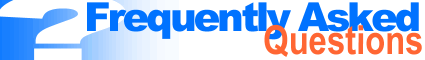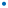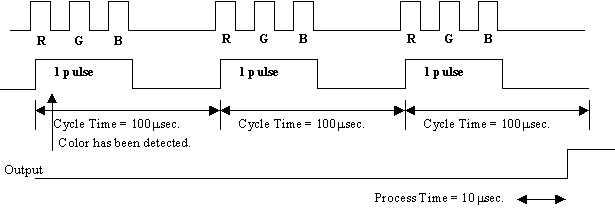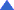• Quick Delivery and Comprehensive Support Most products are in stock and ready for same-day shipping - contact us to get your order started# Sensor QuestionsLocate FAQs by Product LineMeasuring InstrumentsSensors FS Series fiberoptic sensors CZ Series RGB fiberoptic sensors FS-L Series laser fiberoptic sensors PZ2 Series micro photoelectric sensors AP Series digital pressure sensorsPLCsBar Code ReadersVision SystemsSafety Light Curtains

FS Series

1. Can the outputs of the FS-V1 be calibrated separately?

YES, Channel A or B can be calibrated separately by using the UP and Down arrows and adjusting the output point, which is correlated to 100P on the display. This is a good feature in establishing a high and low level for output detection.

2. Can the one-touch calibration be used to separately calibrate the outputs?

YES, Channel A or B can be calibrated separately using the one-touch calibration system. When the button is pushed for the correct calibration sequence, the outputs of A and B are not both affected. If the channels are both in Light ON or Dark ON, the outputs will turn ON and OFF at different time.

3. Can the outputs be separately calibrated externally using the pink wire?

NO, Channel A and B must be calibrated externally together. Reason for this is the internal locking, which does not allow channel A to be locked and not channel B. Both channels get locked; therefore both channels get calibrated.

4. Can the FS-V1 calibrate the specific channel that the display is showing, using its one touch calibration button?

NO, the FS-V1 can only calibrate channels A and B. But if the display is showing channel 6 and the set button is hit, there will be no effect on either channel 6 or the FS-V1 channels A and B. However the set point can be changed by hitting the mode button and using the up and down arrows.

5. What is the maximum amount of FS-T(M)2's that we can connect to the FS-T(M/V)1?

A total of 16 sub units can be connected to the main unit.

6. What is the maximum amount of FS-R3's that we can connect to the FS-R0?

A total of 8 FS-R3's can be connected to the FS-R0; each has 2 inputs and 8*2=16.

7. Can I connect a PNP and a NPN sensor together without any problems?

There will be no problems with stability output or control output, but the external calibration line ( Pink wire ) cannot be used.

8. How many different calibrations are possible with the FS-T/V series amplifier?

The total amount of calibrations possible is 6.

9. How many FS-T's, FS-M(Fine)'s and FS-M(Turbo)'s can we have together without mutual interference?

FS-T = 4
FS-M(Fine) = 4
FS-M(Turbo) = 8

10. If we add on 16 FS-T2's to an FS-T1 amplifier will the response time of the amplifiers decrease?

No, since there is a separate output for each amplifier.

CZ Series

1. What is the Bend Radius and Flex Cycles of the CZ-40/41?
Ans.
CZ-40 - R25mm, 10,000 Flex at 180°
CZ-41 - R15mm, 10,000 Flex at 180°

2. What is the Spectrum or Wavelength that the CZ can detect?
Ans. 350 to 1100 nanometers

3. Why is the beam spot blue and not a mix of all the colors?
Ans. First, all the LED's come on seperately and the reason for the blue color is that it is easiest color for the human eye to see.

4. What is the Cycle and process time of the CZ-K?
Ans.
Cycle time is 100us.
Process time is 100usec when the Response Time is 1ms.
Process time is 10usec when the Response Time is 300us.

5. How long do the Transmitter and Receiver stay ON for during the cycle time?
Ans.
Red is 4.5us.
Green is 9.0us.
Blue is 6.0us.

6. How many Pulses does it take to identify a certain color?
Ans. For 300us the CZ-K needs to see the color for 2 full pulses.
For 1ms the CZ-K needs to see the color for 8 full pulses.7. What is the Repeatability of the CZ-K with the CZ-40/41?
Ans. Repeatability will change depending on the colors. The CZ-41 was checked at a distance of 16mm, looking at the colors Red and Green. The Repeatability was 4um.

8. What is the detectable color Difference?
Ans. deltaE >= 10 ( When the response time is set to 1ms )

9. What are the 3 wavelengths of the LED's?
Ans. RED : 660nm, GREEN : 525nm, BLUE : 470nm

10. What is the response time when switching from Bank to Bank, and when using the KEYENCE KV plc to sample all 8 colors?
Ans. It takes 20ms min. to change from 1 color to another, plus 1ms for response time of sensing the color. To make sure that the color would be seen and switched the KV program takes 25ms for each color bank, for a total of 200ms.

TopFS-L Series

1. What is the beam spot of the FS-L41 and FS-L51?
Ans. For the FS-L41, the beam spot is 4mm or less in dia. and for FS-L51, the beam spot is 6mm or less in dia. Both are nearly parallel beams.

2. How does the Optical system work on the FS-L?
Ans. The laser beam emitted from the laser diode is collected by the lens on the amplifier unit, then transmitted to the glass fiber. The laser beam that has passed through the 50mm dia. glass fiber is converged into a parallel beam by the lens of the fiber unit.

3. Is the receiver and transmitter both made of glass?
Ans. No, the emitter is made of glass, but the receiver is made of 1mm dia. plastic.

4. How do we handle the background effects when ( lets say ) white paper or a mirror-surface object is used?
Ans. When white paper is used as the background, mount the fiber unit 500mm or more from the background. At this distance the background is not detectable. When a mirror-surfaced object is used as the background, however, the background effects detection even if the fiber unit is mounted 500mm or more from the background. When a mirrored-surfaced object is used as the background, tilt the fiber unit slightly so that light reflected off the background does not directly enter the fiber unit.

5. What are the advantages of using a reflector?
Ans. A. Ultra-long detection distance of up to 10m.
B. Easy to align the beam axis, even at a distance, because of the visible beam.
C. 10mm dia. target can be detected.

6. What are the advantages of using the FS-L with optional side-view lens attachment.
Ans. Saves mounting space enabling mounting in any location.

7. What is the Casing, Lens and Sheathing made of for both FS-L's?
Ans. FS-L41 : Casing = Nickel-plated die-cast zinc
Lens = Acrylic resin (PMMA)
Sheath = Vinyl chloride resin (PVC) ( emitter ) and Polyethylene (PE) ( transmitter )

FS-L51 : Casing = Chrome-plated brass
Lens = Acrylic resin (PMMA)
Sheath = Vinyl chloride resin (PVC) ( emitter ) and Polyethylene (PE) ( transmitter )

8. What type of laser is the FS-L and what is the Maximum and Average output laser power of the FS-L?
Ans. The FS-L has a semiconductor laser ( Laser Diode ) with a Peak emission wavelength of 670nm
Maximum output = 3mW, Average output = 0.3mW

9. What is the tensile strength of the fiber unit?
Ans. The fiber unit has a tensile strength of 1kg for 3 seconds maximum.

10. What does the Pink wire do?
Ans. Laser emission control input.

TopPZ2 Series

1. What is the amount of flex cycles for the PZ2-51?
Ans. 1 million times is the flex cycle.

2. What is the wavelength of the PZ2 series?
Ans. 660nm wavelength

3. What are the trimmer caps of the PZ2 series made of?
Ans. They are made of a die-cast zinc alloy, which are, of course, much harder than the plastic caps used in conventional photoelectric sensors.

4. What is the Mean Time Before Failure (MTBF) for the PZ2?
Ans. It is based on the red LED life span, which is 80,000 to 100,000 hours.

5. I've seen a PZ2-41D, what does the "D" stand for in this model's name?
Ans. The "D" stands for the Different Frequency model sensor, which has a different pulsed frequency of light from the transmitter than the PZ2-41. It is used when mounting sensors close together to avoid mutual interference.

6. Which PZ2 models have this "D" option for mutual interference protection?
Ans. There is a PZ2-41(P)D, PZ2-42(P)D, and a PZ2-61(P)D.

7. What is the response time of the different frequency PZ2's?
Ans. The usual response time is 1.5ms, but when changed to a different frequency, the response time is 2ms.

8. Can I use the PZ2 series photoelectric sensor underwater for any length of time?
Ans. The PZ2 series can be used underwater for about 30 minutes, but that is the Maximum time. ( This is because of its IP-67 rating )

9. Does the reflective tape for the PZ2-61, OP-96629, come as a roll of tape or just 1 strip of tape?
Ans. OP-96629 comes as just one strip of tape ( 30mm x 40mm ).

10. What is the maximum temperature for the R1, R2, R3, and R4 reflectors?
Ans. The maximum temperature for the reflectors are 70°C.

11. What is the maximum temperature for OP-92070 and OP-96629 reflective tape?
Ans. The maximum temperature for the reflector tape is 60°C.

12. What is the Power-On reset time for the PZ2?
Ans. 200ms.

TopAP Series

1. Q: What is the IP rating for the AP sensor?
A: The rating of the sensor is IP-40.

2 Q: What is the IP rating of the AP sensor with the OP-31357 protective cover?
A: The rating of the sensor is still IP-40.

3. Q: What does the zero point adjustment do for the AP series sensor?
A: When the zero point adjustment is used (Hold the A key for three seconds), it will zero the display and bring the analog voltage to 1V.

4. Q: What is the linearity of the analog voltage?
A: The linearity of the analog voltage is ±1%.

5. Q: What is the accuracy specification for the AP series?
A: The first step of the accuracy is calculated by multiplying the repeatabilty specification in the instruction manual (0.2%) with the full scale range of pressure of the sensor unit. Here are the numbers as follows.
AP-31K (0 to -14.7 PSI), accuracy is 14.7 x 0.2%= 14.7 x 0.002 = 0.0294 PSI
AP-32K (0 to +14.5 PSI), accuracy is 14.5 x 0.2%= 14.5 x 0.002 = 0.029 PSI
AP-33K (0 to +145 PSI), accuracy is 145 x 0.2%= 145 x 0.002 = 0.29 PSI
AP-34K (14.7 to -14.7 PSI), accuracy is 14.7 x 0.2%= 29.4 x 0.002 = 0.0588 PSI

The resolution of the display has to be figured into the equation as well, so the final accuracy will change to:
AP-31K = 0.04PSI***
AP-32K = 0.04PSI***
AP-33K = 0.4PSI***
AP-34K = 0.06PSI***

***NOTE: These accuracy specifications are also based on the fact that there is no temperature drift and no linearity specification involved.

6. Q: What is the highest amount of pressure that any of the AP sensors can handle before the silicon diaphragm breaks?
A: This specification can be found in the instruction manual under the heading proof pressure. The AP-31, 32, and 34 are resistant up to a pressure level of 5kgf/cm2. The AP-33 is resistant up to a pressure level of 15kgf/cm2. All models of the AP series do not break at a negative pressure, even when there is a total vacuum.

7. Q: What is the response time of the display on the AP-30 series?
A: The response time is set at 200ms, regardless of what the chattering prevention
is set to.

8. Q: What are some gases can the AP-30 Series handle in its port?
A: The AP-30 series can handle the following gases:
Argon
Carbonic Acid gas
Nitrogen
Helium

9. Q: What are some gases can the AP-30 Series not handle in its port?
A: The AP-30 series cannot handle the following gases:
Acetylene gas
Ammonia gas
Chlorine gas
Ozone
Oxygen
Hydrogen
Freon
Natural Gas

10. Q: What are the 5 units that are in the AP-30K series?
A: The 5 units are PSI, PA, inch Hg, kgf/cm2, and bar.
For positive pressure the units are: PSI, bar, Kgf/cm2, and Pa
For negative pressure the units are: mmHg, inchHg, Pa, and bar

11. Q: What is the most negative pressure that the AP can handle?
A: The AP sensor can handle -760 Torr (-760 mm Hg) or -14.7 PSIG, but in fact
the condition of this perfect vacuum does not exist in the AP because of air leakage.

12. Q: I see an FFF on my display, what does this mean?
A: You have reached 110% of the F.S. reading of the AP sensor that you are using.

13. Q: I see a -FFF on my display, what does this mean?
A: This means you have reached the -15% of the F.S. reading on the AP sensor that you are using.

14. Q: What is the range of the analog output for the AP-34K compound pressure type?
A: The AP-34 produces 1V at a positive pressure of +760mmHg, 3V at atmospheric pressure and 5V at a negative pressure of -760mmHg.

15. Q: Is it safe to apply negative pressure to the positive pressure type?
A: Yes, all models of the AP series do not break at negative pressure.

16. Q: How long is the life of the diaphragm?
A: One hundred million times. As a comparison, the mechanical Bourdon tube is
one million times.

17. Q: What if you wanted to set-up a target/no target situation on a seating
confirmation application; where the part sits on a air blower and stops the air flow completely when it is seated correctly, and allows some air out when it is not seated corectly? Also, the actual pressure for each situation is not known.

A: The solution would have to be Auto-tuning mode (F-1). This allows you to take in both the target situation pressure and the no target situation pressure, and get a preset that will be your trigger point. The points will be A - your highest pressure, then little b - your lowest pressure, and C- the midpoint of the two pressures you brought in (changeable). There is also, a Stable High - SH, and a Stable Low - SL that will automatically be calculated within the AP. So, if the correct seating pressure was 13 PSI (A) and the incorrect seating pressure was 9 PSI (b), the AP would take the midpoint of 11 and use that as the preset value C. SH would be the midpoint of A and C (SH = 12 PSI) and SL would be calculated as the midpoint of b and C (SL = 10 PSI). With this set-up, any pressure above 11 PSI (C) will turn on output 1, and anything outside of SH and SL will turn on output 2.

18. Q: What if I had an application where I needed to make sure that the pressure reached 10 PSI (output 1 turned on) and then did not drop below 7 PSI. If it did drop below 7 PSI, output 2 would turn on as an alarm output ?
A: The best way to do this is to use the Hysteresis mode (F-2) of the AP-32 or AP-33. You would set the H value in this mode to 10 PSI and the little h value to 3 PSI (allowable hysteresis). This will set-up a 10 as your H , and the (10 - 3 = 7) value for your allowable hysteresis. When above 10 PSI, output 1 will turn on and stay on until you drop below 7 PSI, where output 2 will turn on.

19. Q: Can I set up a high, low, and go with the AP sensor's output, and what would I use?
A: You certainly can, and the best way to do it is to use two independent output
Mode (F-3). Let's say you wanted to have below 3PSI, a LOW, between 3 and
5 PSI, a GO, and above 5PSI a HIGH. What you would do is set A for 5, little
b for 3, and use the outputs in the following manner:
With neither of the outputs on - LOW
With just output 2 on (above little b) - GO
With both output 1 (above A) and output 2 on (above little b) - HIGH

20. Q: Can I set-up the AP's output 1 to trigger between 4 and 8 PSI , and output 2 to turn on when I have a stable reading within this window?
A: Yes, by using Window mode (F-4) on your AP sensor. What you would do is set-up the H to be 8 and the L to be 4. When you are inside of the H and L values, Output 1 will turn on, and if you are within the stability levels calculated automatically inside the AP sensor; Stability High (SH) = H - (H-L)/4 = 7 PSI, and Stability Low (SL) = L + (H-L)/4 = 5 PSI, output 2 will turn on.

21. Q: What is the highest temperature that the AP-30 Series can handle?
A: The highest amount of temperature that the AP-30 Series can handle is 50°C.

22. Q: Why is the analog output of the AP so important?
A: This analog option gives you readings for the entire pressure range, where the control outputs can give you at most two readings (basically two presets that you create in independent output mode for example). You can bring the analog readings from the AP into a KV-AN6 and actually see the entire range in the KV, and from there set-up several presets within the KV itself to send out various outputs.

23. Q: I received a preset value of 6.4 PSI for my C value in Auto-tuning mode, and I was wondering if this preset can be changed to 7 PSI?
A: The quickest way would be to hit the enter key while in measuring mode, then scroll through the A value and the b value by hitting the enter key twice. The C value should appear blinking with the preset of 6.4 PSI. If you hit the up and down arrow keys, the value can be changed; change it to 7 PSI

24. Q: Can I change the color of the display so that red is green and green is red?
A: Yes, by choosing the NC option this will change the display color, as well as make the outputs normally closed.

25. Q: What is the amount of hysteresis of the AP-30 series sensors?
A: The amount of hysteresis in the AP-30 series (when used in modes F-1, F-2, and F-4) is automatically 0.5% of full scale for all AP's. On the other hand, there is no automatic hysteresis specification in Hysteresis mode (F-3) for all of the sensors. The hysteresis of the AP sensor in Hysteresis mode F-3 will be the little h value you give it in setup.

26. Q: What is the air leakage specification of the input port of the AP-30K Series?
A: There is no air leakage in the sensor's input port to the top of the silicon
diaphragm. The sensor is though ventilated on the other side of the diaphragm to the atmosphere (in order to measure gage pressure).

27. Q: What does proof pressure mean?
A: This is the maximum "spike" of pressure that the sensor can handle. For
example, the AP-31's proof pressure is 72.5 PSI. Any pressure above this specification of 72.5 PSI can have adverse affects on the sensor's operation.

28 Q: Can I get the peak value that my AP experienced in one day?
A: Yes, all you need to do is hold the key on the upper right of the display. By holding this "UP" key, the AP's display will show the highest value it saw during the day.

29. Q: Can I get the bottom value that my AP experienced in one day?
A: Yes, all you need to do is hold the key on the lower right of the display. By holding this "DOWN" key, the AP's display will show the lowest value it saw during the day.

30. Q: How can I reset the peak and bottom value of my AP sensor?
A: One way is to hold the and then press in measurement mode. The other is to turn the unit off and turn it back on.

31. Q: Can we do differential pressure with the AP-30K Series?
A: No, differential pressure is based on two separate pressures coming into a sensor and the difference between the two pressures is your final pressure. The only pressure that we can use the AP-30K series for is Gage Pressure, which is based on the pressure you received minus atmospheric pressure.

32. Q: Is the AP-30K series explosion proof ?
A: No, the AP-30K series does not have an explosion-proof structure. Do not use the AP for the detection of flammable gases.

33. Q: What is the maximum load that we can put on the output lines (black and the white line) of the AP-30K?
A: The maximum that we can put on either of the output lines is 40V max at 100mA max. This maximum load specification means that this is the highest amount of current and voltage that I can put across a resistance ( like a light bulb or a resistor for example) without destroying the transistor of the AP-30K sensor.

Top